# 1116. Data Structure - LRU CacheLRU and Cache

Implement Least Recently Used(LRU) cache.

## 1. LRU

### 1.1 LRU Cache Algorithm

Least Recently Used(LRU) cache algorithm keeps recently used items near the front of cache. Whenever a new item is accessed, the LRU places it at the head of the cache. When the cache reaches to its capacity, items that have been accessed less recently will be removed starting from the end of the cache.

### 1.2 How It Works?

The LRU cache provides two methods: put and get.

• put(key, value) - Set or insert the value if the key is not already present. When the cache reached its capacity, it should invalidate the least recently used item before inserting a new item.
• get(key) - Get the value of the key and move this item to the head of the cache. If the key doesn’t exist in the cache, return -1.

The following diagram illustrates how LRU works.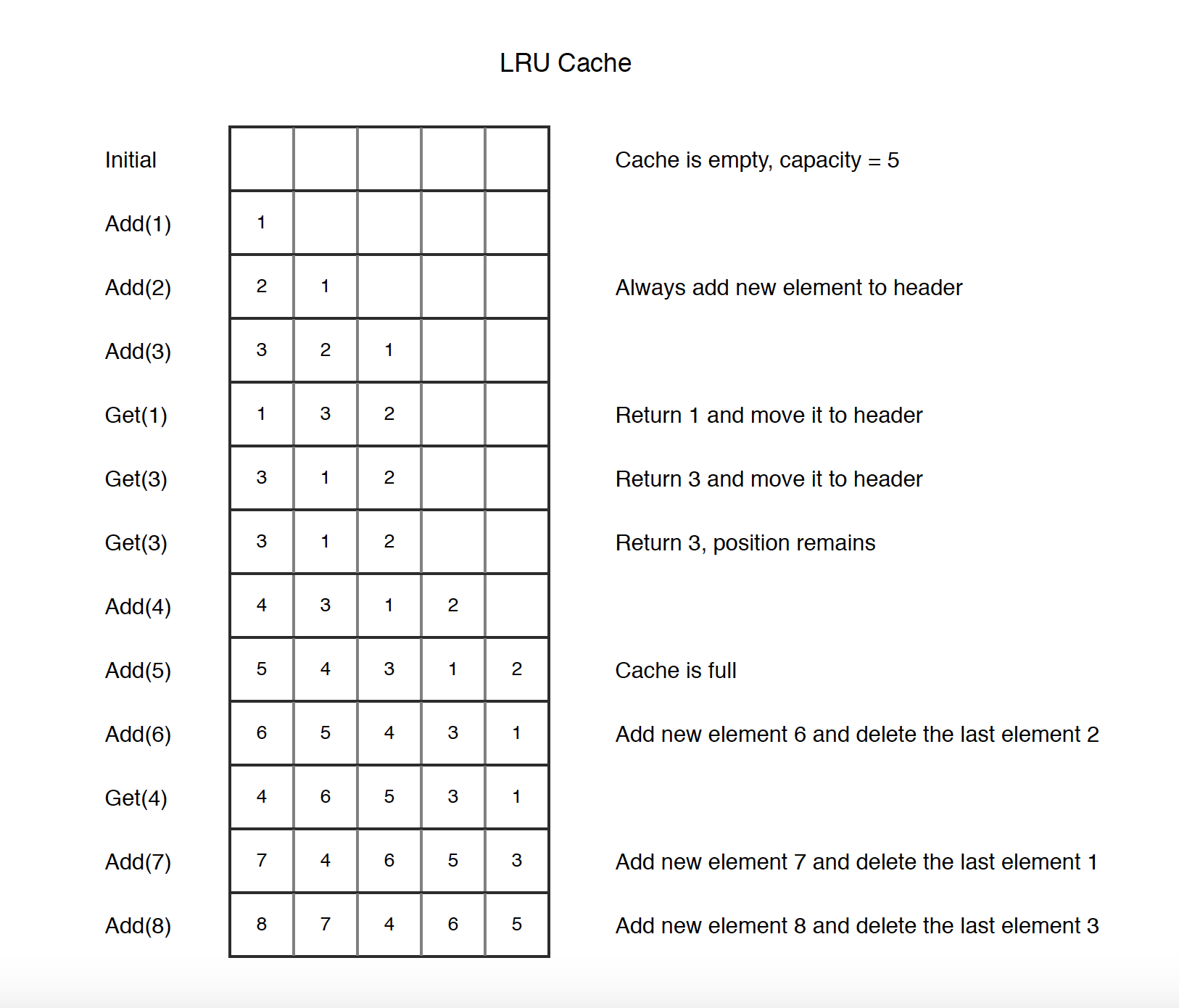## 2. Implementation

### 2.1 Data Structure

Generally, LRU algorithm is implemented with HashMap and Doubly Linked List.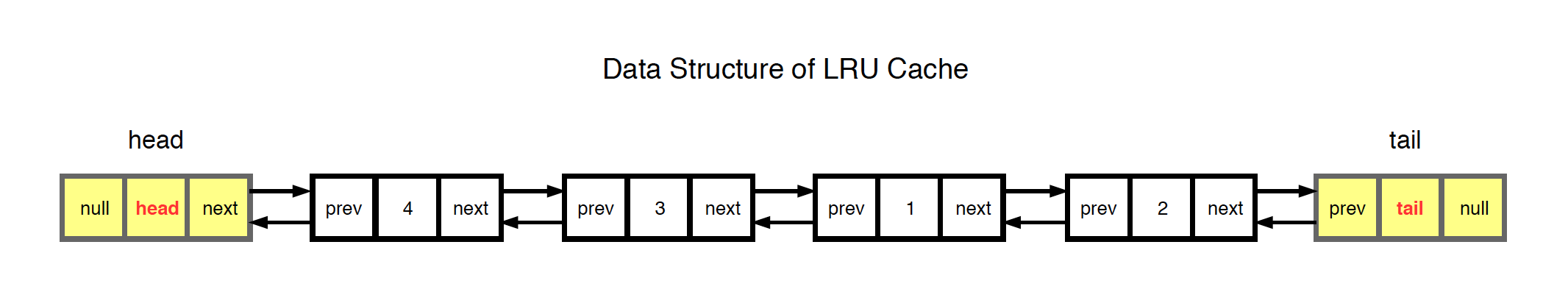• The head and tail nodes don’t store any data. They are created just for conveniently manipulating the linked list.
• Nodes between the head and tail nodes are used to store data, each node for one value. Every node has two pointers, pointing to the previous and the next nodes. They are connected to each other.
• Nodes near the tail are least recently accessed. They will be removed if cache reaches to its capacity.

### 2.2 Operations On LRU

1) Initialization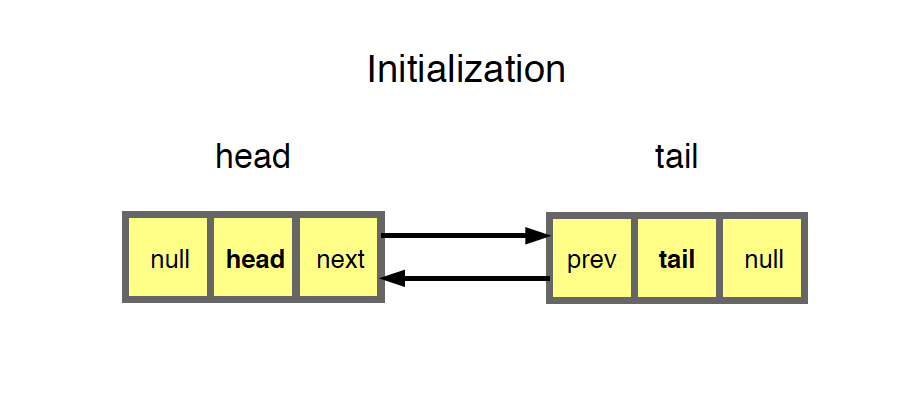• Only two dummy nodes, head and tail.
• Notice that there is another HashMap which stores the value-node pair.

2) Put (Cache is not full)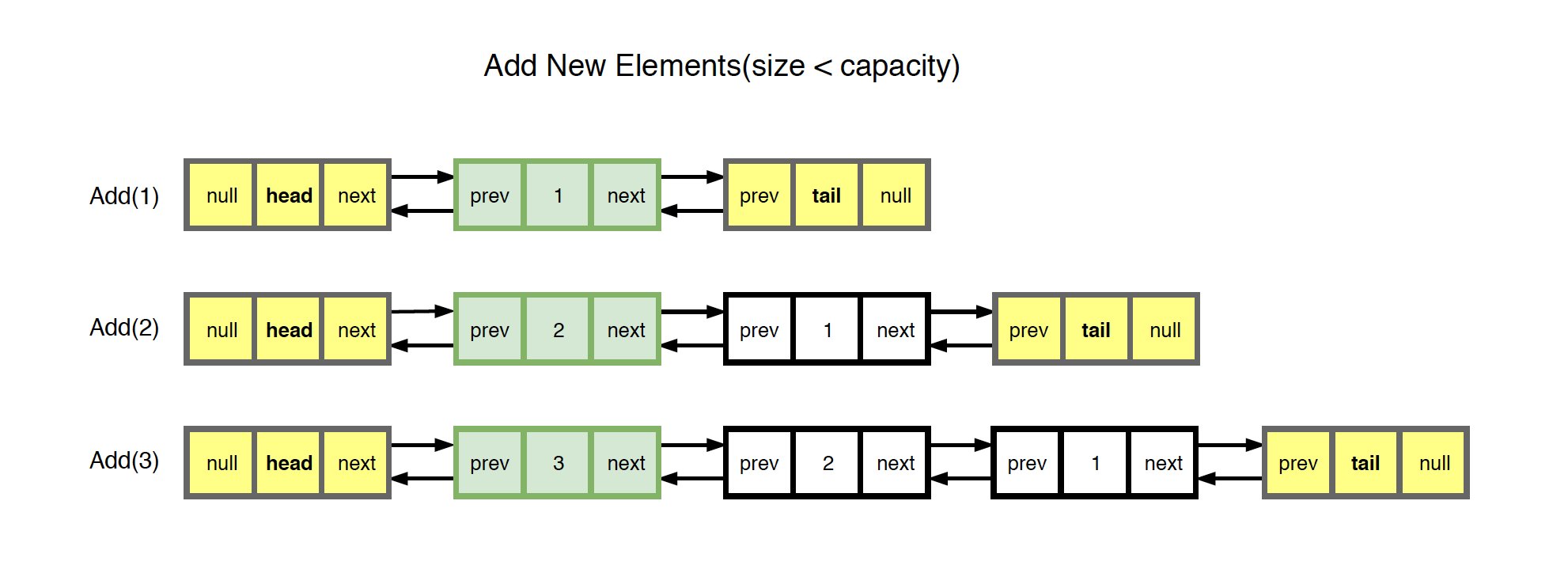• Create new node for the given value and insert it to the head of the linked list.
• Put the new node to HashMap with the given value as key.
• Size is increased by one.

3) Put (Cache is full)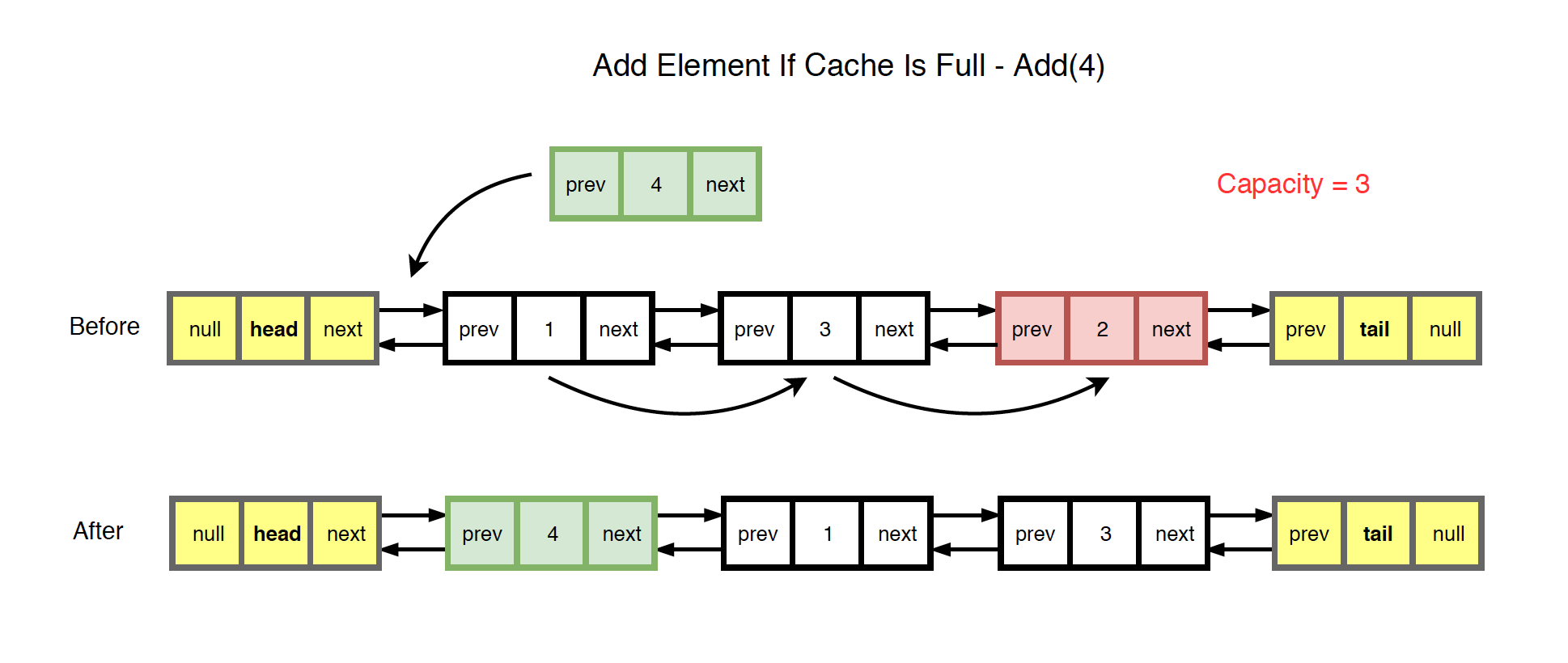• Remove the last element(The one tail.prev is pointing) from the list.
• Create new node for the given value and insert it to the head of the linked list.
• Put the new node to HashMap with the given value as key.
• Size remains unchanged.

4) Get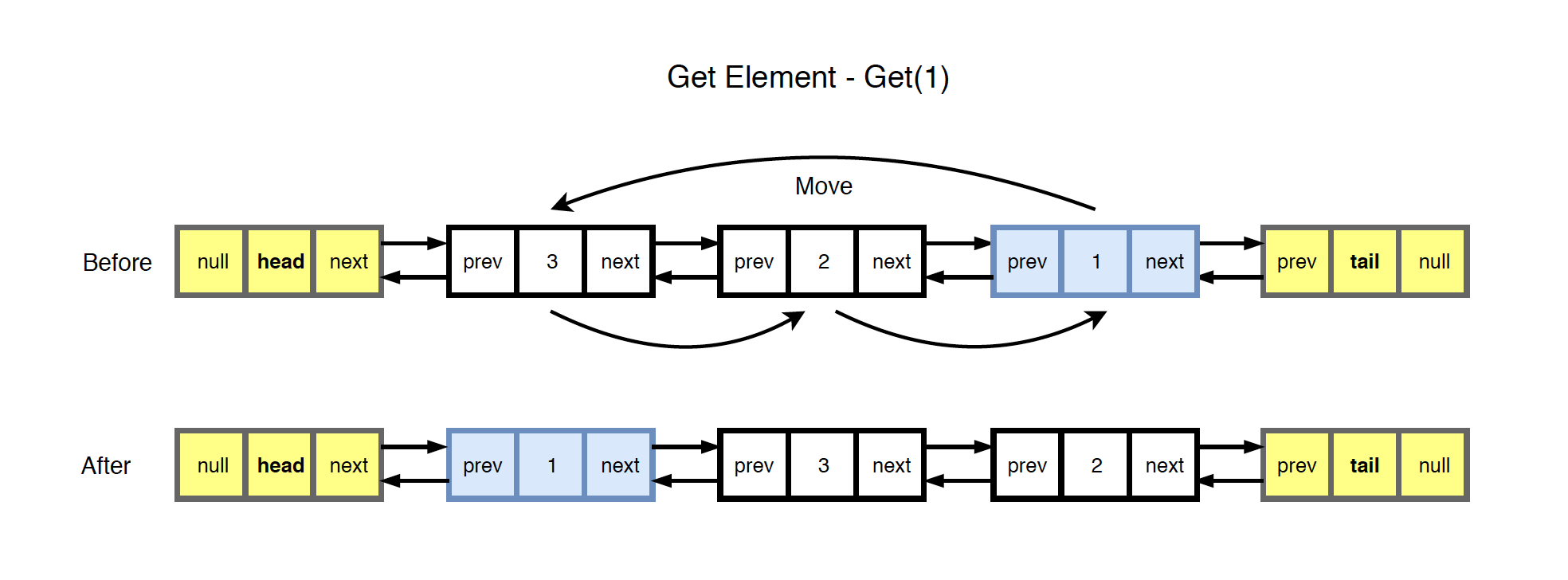• Find the given value in HashMap.
• If the corresponding node is not at the head position of the linked list, move it to head.
• Update the tail pointers accordingly.
• Return the value.

### 2.3 Implementation with Custom Node

The following code is the implementation of LRU based on custom nodes. The node is defined as follows.

public class Node {
public int key;
public int val;
public Node prev;
public Node next;

public Node(int key, int val) {
this.key = key;
this.value = val;
this.prev = null;
this.next = null;
}
}


Following is the LRU class which implements the put() and get() methods.

public class LRU {
private int capacity;
private HashMap<Integer, Node> map; // key, node
private Node head;                  // The latest accessed element
private Node tail;                  // The least recently used element

public LRU(int capacity) {
this.capacity = capacity;
this.map = new HashMap<>();
this.tail = new Node(-1,-1);
}

public void put(int key, int val) {
if (map.containsKey(key)) {
Node node = map.get(key);
node.val = val;
node.prev.next = node.next;
node.next.prev = node.prev;
return;
}

if (map.size() == capacity) {
map.remove(tail.prev.key);
tail.prev = tail.prev.prev;
tail.prev.next = tail;
}

Node node = new Node(key, val);
map.put(key, node);

// move new node to head
}

public int get(int key) {
if (!map.containsKey(key)) {
return -1;
}

// remove current
Node node = map.get(key);
node.prev.next = node.next;
node.next.prev = node.prev;

// move current node to head

return node.val;
}

node.next.prev = node;
}
}


Time complexity:

• put() - $O(1)$
• get() - $O(1)$

Space complexity:

• $O(n)$, 2*N, N is the number of nodes

### 2.4 Implementation with Deque

Instead of creating the doubly linked list by hand, we can use Deque directly in Java. The following LRUDeque class implements LRU with Deque. The efficiency of the get() method may be $O(n)$ in the worst case.

public class LRUDeque {
private int capacity;
private HashMap<Integer, Integer> map; // key, value
private Deque<Integer> deque;          // key

public LRUDeque(int capacity) {
this.capacity = capacity;
this.map = new HashMap<>();
}

public void put(int key, int val) {
if (map.containsKey(key)) {
map.put(key, val);
// remove current
deque.remove(key); // equivalent to removeFirstOccurrence(), performance issue, O(n)
return;
}

if (map.size() == capacity) {
// remove the least recently used element from map and deque
map.remove(deque.removeLast());
}

map.put(key, val);
}

public int get(int key) {
if (!map.containsKey(key)) {
return -1;
}

// remove current
deque.remove(key); // equivalent to removeFirstOccurrence(), performance issue, O(n)

return map.get(key);
}
}


Time complexity:

• put() - $O(1)$
• get() - $O(n)$

Space complexity:

• $O(n)$, 2*N, N is the number of nodes

### 2.5 Testing

Create an instance of LRU class and call put() and get() methods. The change of the list is described in the inline comments.

LRU lru = new LRU(5); //capacity = 5
lru.put(1,1); // values = 
lru.put(2,2); // values = [2,1]
lru.put(3,3); // values = [3,2,1]
lru.get(1);   // values = [1,3,2], return 1
lru.get(3);   // values = [3,1,2], return 3
lru.get(3);   // values = [3,1,2], return 3
lru.put(4,4); // values = [4,3,1,2]
lru.put(5,5); // values = [5,4,3,1,2], cache is full
lru.put(6,6); // values = [6,5,4,3,1], remove least recently visited element, 2
lru.get(4);   // values = [4,6,5,3,1], return 4
lru.put(7,7); // values = [7,4,6,5,3], remove 1
lru.put(7,2); // values = [2,4,6,5,3], element with key=7 is updated
lru.get(7);   // keys = [7,4,6,5,3], values = [2,4,6,5,3], return 2
lru.put(3,9); // keys = [3,7,4,6,5], values = [9,2,4,6,5], move key=3 to head
lru.get(3);   // keys = [3,7,4,6,5], values = [9,2,4,6,5], return 9